# 知识回顾

在正式说MCMC方法实现之前，我们要先来回顾一下马尔科夫链的一系列知识。

$\pi$是方程$\pi P = \pi$的唯一非负解

$\pi$称为马氏链的平稳分布。

则$\pi(x)$是马氏链的平稳分布，上式被称为细致平稳条件(detailed balance condition)。其实这个定理是显而易见的， 因为细致平稳条件的物理含义就是对于任何两个状态$i,j$, 从$i$转移出去到$j$而丢失的概率质量，恰好会被从$j$转移回$i$的概率质量补充回来， 所以状态$i$上的概率质量$\pi(i)$是稳定的，从而$\pi(x)$是马氏链的平稳分布。数学上的证明也很简单，由细致平稳条件可得

由于$\pi$是方程$\pi P = \pi$的解，所以$\pi$是平稳分布。

# MCMC算法-Metropolis-Hastings采样

假设我们已经有一个转移矩阵为$Q$马氏链（$q(i,j)$表示从状态$i$转移到状态$j$的概率，也可以写为$q(j|i)$或者$q(i\rightarrow j)$, 显然，通常情况下$p(i) q(i,j) \neq p(j) q(j,i)$也就是细致平稳条件不成立，所以$p(x)$不太可能是这个马氏链的平稳分布。我们可否对马氏链做一个改造， 使得细致平稳条件成立呢？比如，我们引入一个$\alpha(i,j)$, 我们希望

取什么样的$\alpha(i,j)$以上等式能成立呢？最简单的，按照对称性，我们可以取

于是$(*)$式就成立了。所以有

于是我们把原来具有转移矩阵$Q$的一个很普通的马氏链，改造为了具有转移矩阵$Q'$的马氏链，而$Q′$恰好满足细致平稳条件， 由此马氏链$Q′$的平稳分布就是$p(x)$

在改造$Q$的过程中引入的$\alpha(i,j)$称为接受率，物理意义可以理解为在原来的马氏链上，从状态$i$$q(i,j)$的概率转跳转到状态 $j$的时候，我们以$\alpha(i,j)$的概率接受这个转移，于是得到新的马氏链$Q′$的转移概率为$q(i,j)\alpha(i,j)$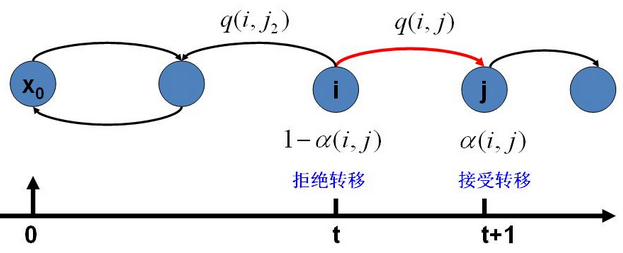假设我们已经有一个转移矩阵$Q$(对应元素为$q(i,j)$), 把以上的过程整理一下，我们就得到了如下的用于采样概率分布$p(x)$的算法。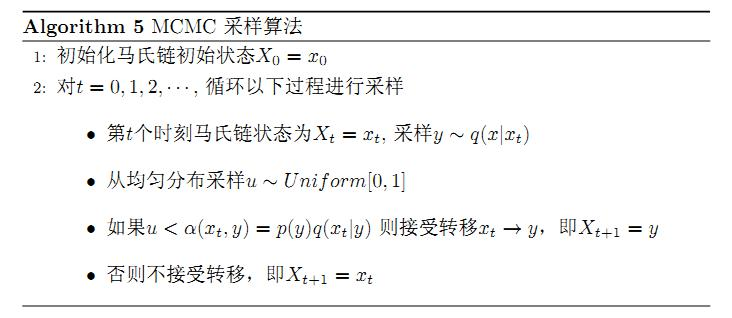上述过程中$p(x),q(x\vert y) 说的都是离散的情形，事实上即便这两个分布是连续的，以上算法仍然是有效，于是就得到更一般的连续概率分布$p(x)$的采样算法，而$q(x\vert y)就是任意一个连续二元概率分布对应的条件分布。

以上的 MCMC 采样算法已经能很漂亮的工作了，不过它有一个小的问题：马氏链Q在转移的过程中的接受率$\alpha(i,j)$可能偏小， 这样采样过程中马氏链容易原地踏步，拒绝大量的跳转，这使得马氏链遍历所有的状态空间要花费太长的时间，收敛到平稳分布$p(x)$的速度太慢。 有没有办法提升一些接受率呢?

假设$\alpha(i,j)=0.1, \alpha(j,i)=0.2$，此时满足细致平稳条件，于是

上式两边扩大5倍，我们改写为

我们提高了接受率，而细致平稳条件并没有打破！这启发我们可以把细致平稳条件$(**)$式中的$\alpha(i,j),\alpha(j,i)$同比例放大， 使得两数中最大的一个放大到1，这样我们就提高了采样中的跳转接受率。所以我们可以取

于是，经过对上述MCMC 采样算法中接受率的微小改造，便得到了Metropolis-Hastings 算法。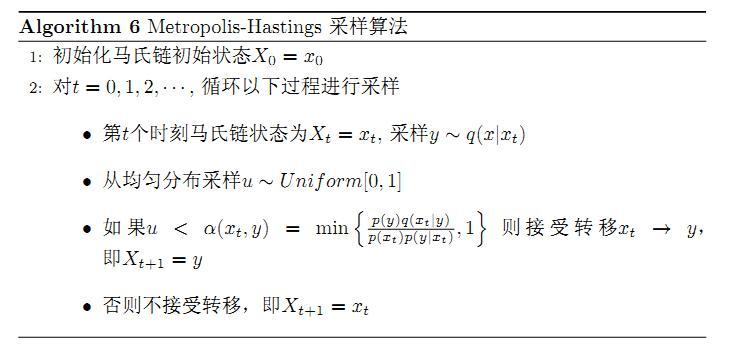# MCMC算法-Gibbs采样

对于高维的情形，由于接受率$\alpha$的存在(通常$% $), 以上 Metropolis-Hastings 算法的效率不够高。 能否找到一个转移矩阵$Q$使得接受率$\alpha=1$呢？我们先看看二维的情形，假设有一个概率分布$p(x,y)$，考察$x$坐标相同的两个点 $A(x_1,y_1),B(x_1,y_2)$，可得

基于以上等式，我们发现，在$x=x_1$这条平行于$y$轴的直线上，如果使用条件分布$p(y\vert x1)$做为任何两个点之间的转移概率， 那么任何两个点之间的转移满足细致平稳条件。同样的，如果我们在$y=y_1$这条直线上任意取两个点$A(x_1,y_1),C(x_2,y_1)$,也有如下等式 $p(A)p(x_2|y_1) = p(C)p(x_1|y_1)$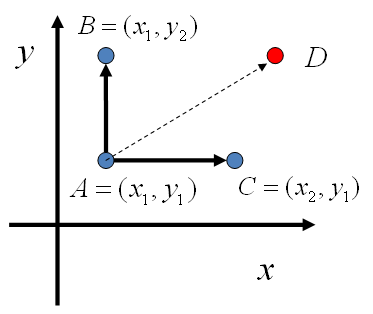于是我们可以如下构造平面上任意两点之间的转移概率矩阵$Q$

有了如上的转移矩阵$Q$, 我们很容易验证对平面上任意两点$X,Y$, 满足细致平稳条件

于是这个二维空间上的马氏链将收敛到平稳分布$p(x,y)$。而这个算法就称为 Gibbs Sampling 算法,是 Stuart Geman 和Donald Geman 这两兄弟于1984年提出来的，之所以叫做Gibbs Sampling 是因为他们研究了Gibbs random field, 这个算法在现代贝叶斯分析中占据重要位置。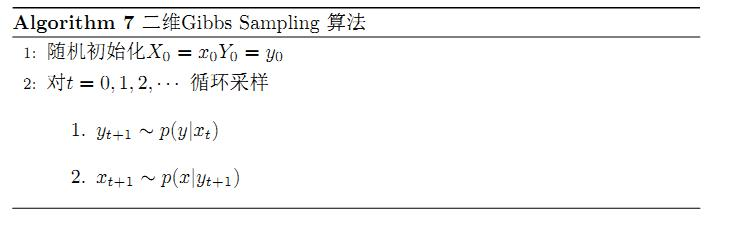以上采样过程中，如图所示，马氏链的转移只是轮换的沿着坐标轴$x$轴和$y$轴做转移，于是得到样本 $(x_0,y_0), (x_0,y_1), (x_1,y_1), (x_1,y_2),(x_2,y_2), \cdots$马氏链收敛后，最终得到的样本就是$p(x,y)$的样本， 而收敛之前的阶段称为 burn-in period。

上述的二维Gibbs采样可以拓展到$n$维。

# 总结

在贝叶斯公式得到的后验分布将变得非常复杂时，马尔科夫链蒙特卡罗方法帮助解决了此类问题。Metropolis-Hastings采样和Gibbs采样是MCMC的两大算法， 围绕着贝叶斯和此类采样问题整体的思路基本上就是这些。